# 2018-05-07 Basic

A vertex of one square is pegged to the center of an identical square, and the overlapping area is blue. One of the squares is then rotated about the vertex and the resulting overlap is red.

Which area is greater?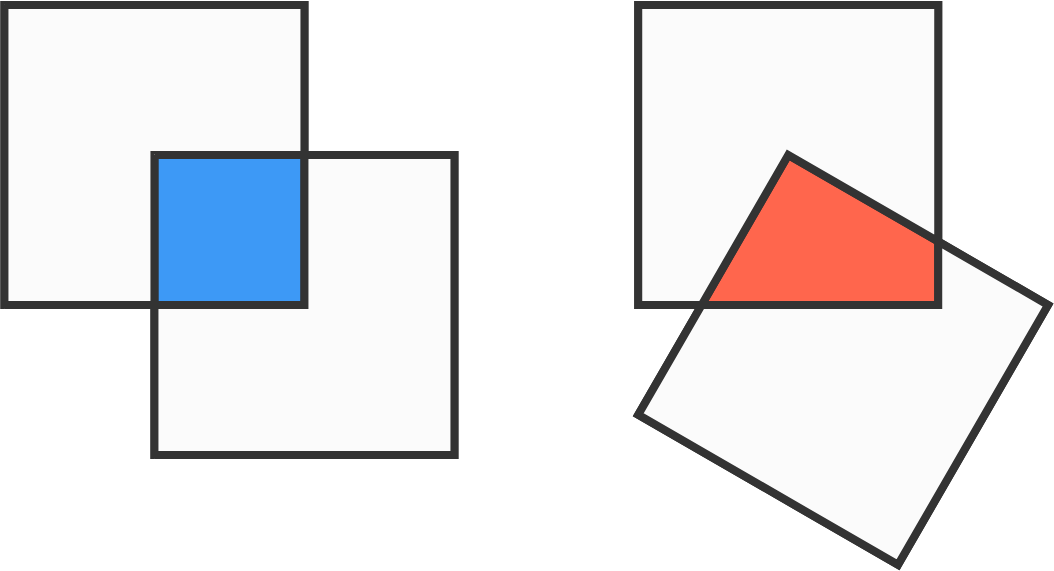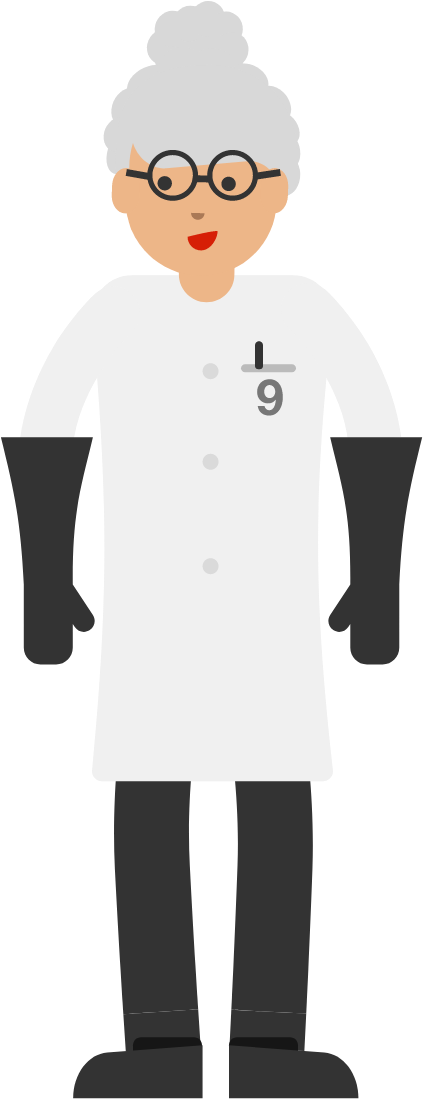Dr. Frankenine (cousin of Dr. Frankenthree) took positive integers, reversed their digits, and subtracted the two numbers. She noticed all the results were divisible by 9: \begin{aligned} {\color{#3D99F6}83}-{\color{#20A900}38} &= 45 \\&= 9 \times 5 \\\\ {\color{#3D99F6}113}-{\color{#20A900}311} &= -198 \\&= 9 \times (-22) \\\\ {\color{#3D99F6}1431} -{\color{#20A900}1341} &= 90 \\&= 9 \times 10 \\\\ {\color{#3D99F6}44344} -{\color{#20A900}44344} &= 0 \\&= 9 \times 0. \end{aligned} Are the numbers Dr. Frankenine creates in this way always multiples of 9?

Note: This puzzle is in base 10.

Consider the following sequence of events:

$\hspace{2mm}$ 1. Start with a full gasoline tank.
$\hspace{2mm}$ 2. Use half the gas.
$\hspace{2mm}$ 3. Re-fill the tank with gasoline until it's full.
$\hspace{2mm}$ 4. Use a quarter of the gas.
$\hspace{2mm}$ 5. Re-fill the tank with gasoline until it's full.

What percentage of the gas in the tank after step (5) was originally there in step (1)?

Note: Assume perfect mixing between steps. Enter your answer as a percentage. For example, 58.4% would be entered as 58.4.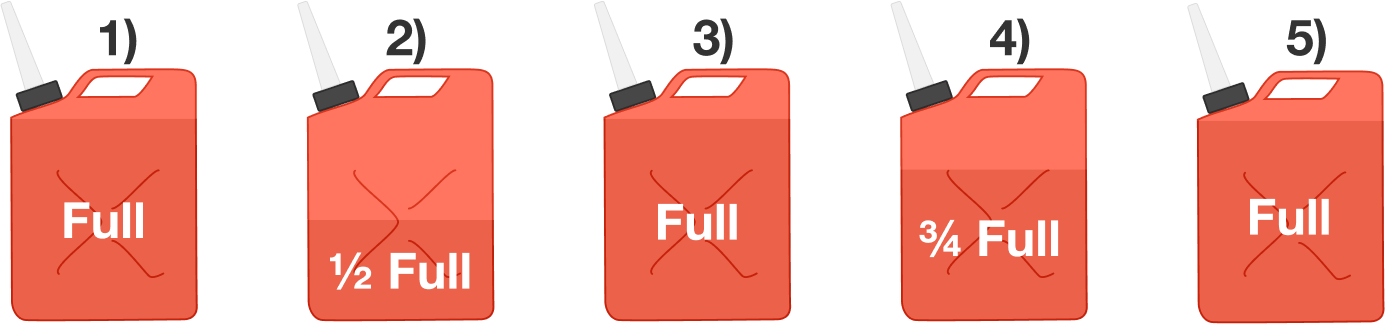Sam is standing at one end of a $\SI{10}{\meter}$ board on an ice floor. The board’s mass is $\SI{30}{\kilo\gram}$ and Sam weighs $\SI{50}{\kilo\gram}.$

What distance, with respect to the ice on the ground, does Sam travel if he walks to the other end of the board?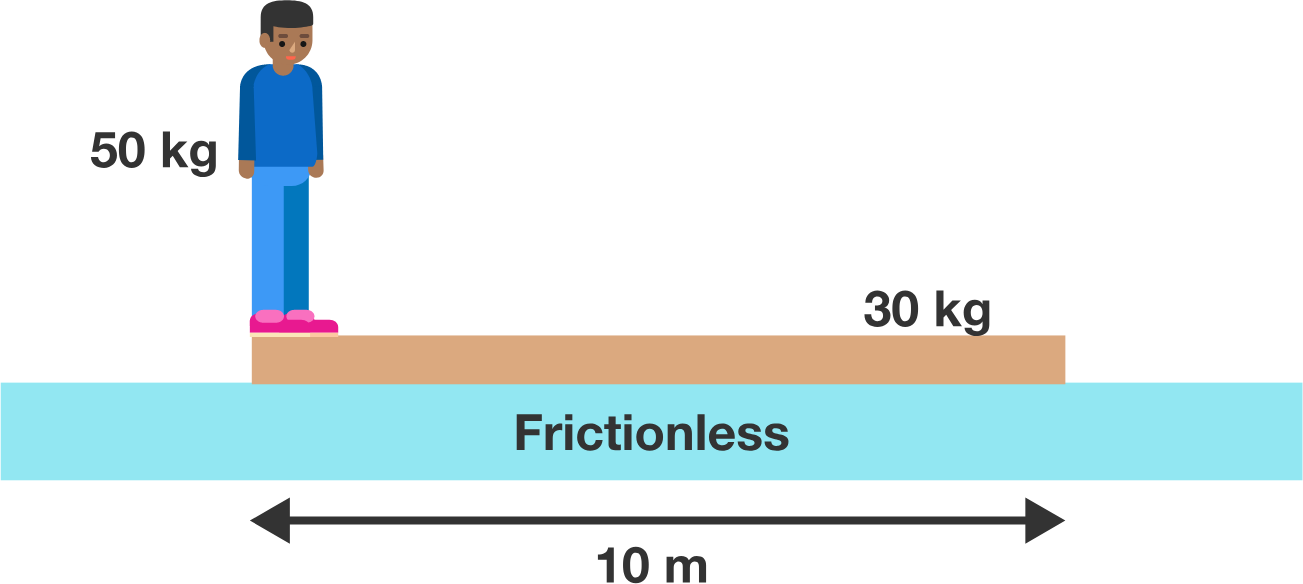Assumptions: for simplicity, assume that there is no friction between the ice and the board.

How many squares can you make using points on this $5\times 5$ grid as their vertices?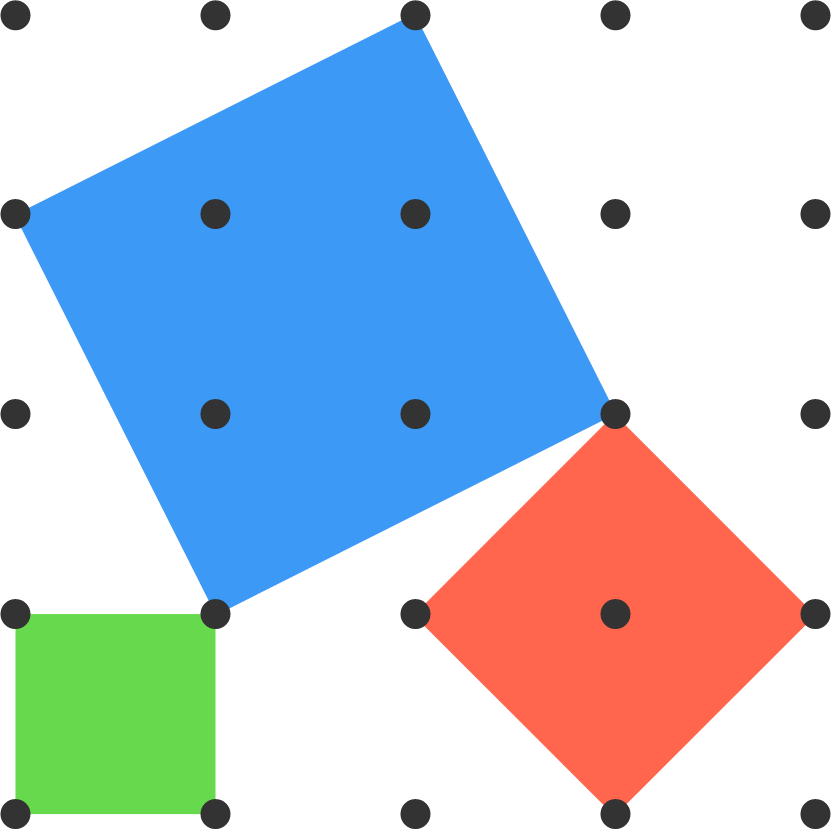Three possible squares are shown.

Note: Some of the squares can be the same size, and they can overlap one another.

×

Problem Loading...

Note Loading...

Set Loading...